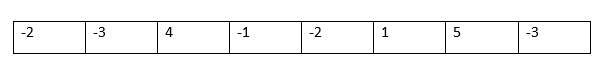# Largest Sum Contiguous SubarrayThis is one of the mostly asked question during interviews. Here interviewer loves to see a linear algorithmic approach of yours. Yes, you can find the maximum sum of elements in linear time using a single traversal of the array. Here we will be displaying the largest sum of the contiguous subarray.

We have to find the sum of a contiguous subarray in an array of numbers which has the largest sum. This can be solved using Kadane’s Algorithm which is a Dynamic Programming approach.

## Input FormatFirst-line containing an integer value N.

Second-line containing an array of size N  or second-line containing N integers.

## Output FormatPrint a single integer value which denotes the largest sum of the contiguous subarray.

## Constraints• 1<=N<=100000
• -10^9<=a[i]<=1e9, where a[i] denotes the element of the array.

## Approach 1 for Largest Sum Contiguous Subarray by using Kadane’s AlgorithmYou have an array of numbers. Numbers can be positive and negative and in random order. You have to give a set of continuous elements from an array whose sum is maximum. For example, if you have an array of following numbers-

Last Stone Weight

Input: -2, -3, 4, 1, -2, 1, 5, -3

Output: 9

We will use a dynamic programming approach. It is also known as Kadane’s algorithm. Also, we will print the subarray with the maximum sum.### Algorithm for Largest Sum Contiguous Subarray

1. Take input N and then an array a[] of size N.

2. Take two variables prev=a and sum= a.

3. For i in range 0 to N-1:

a. prev = max( prev+a[i], a[i] )

b. sum = max( sum, prev )

3. Print the value sum.

### C++ Program

```#include <bits/stdc++.h>
using namespace std;
int main()
{
int n;
cin>>n;
long long int a[n];
for(int i=0;i<n;i++)
{
cin>>a[i];
}
long long int prev=a,sum=a;
for(int i=0;i<n;i++)
{
prev=max(prev+a[i],a[i]);
sum=max(sum,prev);
}
cout<<sum<<endl;
return 0;
}```

### JAVA Program

```import java.util.Arrays;
import java.util.Scanner;
public class Main {
public static void main(String[] args) {

int n;
Scanner s = new Scanner(System.in);
n = s.nextInt();
int a[] = new int[n];
for(int i=0;i<n;i++)
{
a[i]=s.nextInt();
}
int maxSum=0,sum=0;
int i;
for(i=0;i<n;i++)
{
sum=sum+a[i];
if(sum<0)
{
sum=0;
}
else if(sum>maxSum)
{
maxSum=sum;
}
}
System.out.println(maxSum);
}
}```
```8
-2 -3 4 1 -2 1 5 -3```
`9`

### Complexity Analysis for Largest Sum Contiguous Subarray

#### Time Complexity

O(N) where N is the size of the given array. Here we just traverse the array and update the value of the variables and at the last print the answer.

#### Space Complexity

O(1) because we don’t use any auxiliary space here. We just store the current sum and maximum sum in two variables.

## Approach 2 for Largest Sum Contiguous Subarray by using Prefix Sum ArraySimply use Brute Force way by obtaining all the subarray sums and find the maximum among them.

### Algorithm for Largest Sum Contiguous Subarray

1.    Compute the prefix sum array-

Find Three Element From Different Three Arrays Such That a + b + c = sum

For i in range 0 to N-1:

if i == 0 then:

presum[i] = a[i]

else

presum[i] = presum[i-1]+a[i]
2.    Now, for each i and j where i varies from 0 to N-2 and j varies from i to N-1 compute the sum of the subarray as presum[j]-presum[i-1]
3.    Store the maximum sum of the subarray in sum variable.

At the end when we compute all the N*(N+1)/2 subarrays we get the maximum.

### C++ Program

```#include <bits/stdc++.h>
using namespace std;
int main()
{
int n;
cin>>n;
long long int a[n];
long long int presum[n];
for(int i=0;i<n;i++)
{
cin>>a[i];
if(i==0)
{
presum[i]=a[i];
}
else
{
presum[i]=a[i]+presum[i-1];
}
}
long long int ans=0;
for(int i=0;i<n-1;i++)
{
for(int j=i;j<n;j++)
{
long long int sum=0;
if(i==0)
{
sum=presum[j];
}
else
{
sum=presum[j]-presum[i-1];
}
ans=max(ans,sum);
}
}
cout<<ans<<endl;
return 0;
}```
```8
-10 5 6 3 -16 9 8 -15```
`17`

### Complexity Analysis for Largest Sum Contiguous Subarray

#### Time Complexity

O(N*N) where N is the size of the array. Here we find the sum of all the subarrays and store the maximum of them. For finding the sum of each subarray we need to use nested for loop which lead us to O(N*N) time complexity.

#### Space Complexity

O(N) because we create a prefix sum array to store the sum and by the use of this array we find the sum of a subarray in O(1) time only.

Subarray with the maximum sum is one of the most asked questions in product-based companies during technical interviews. Try to practice and explain the logic of Kadane’s algorithm when they ask you to explain.

References